# NCERT Solutions For Class 10 Maths Chapter 10

## NCERT Solutions Class 10 Maths Circles Tangents Perpendicular

### Ncert Solutions For Class 10 Maths Chapter 10 PDF Free Download

We at BYJU’S provide class 10 Maths Chapter 10 Circles NCERT Solutions and are available in PDF format for free. Circles is one of the most important topic for the students of class 10 as well as for the various competitive board examinations. Students can either use this solution online or download the PDF files and prepare for their exams. These circles class 10 ncert solutions pdf are very detailed and prepared by subject our experts. You can see step-by-step solved solutions to all the questions existing in class 10 maths NCERT textbook syllabus (2018-2019).

This Circles chapter of class 10 maths deals with the various types of questions such as finding the angles, length of a chord, length of a tangent, tangent drawn from a point to a circle, etc., which should be practiced thoroughly to have a better learning experience and also to excel in the examination. BYJU’S study materials include different types of exemplar problems of class 10 on circles, radii of a circle, the diameter of a circle, finding the length of the segment and lot more.

### NCERT Solutions Class 10 Maths Chapter 10 Exercises

1. In the figure, PQ is a chord of a circle and PT is the tangent at P such that ∠QPT = 60°. Then find the measure of ∠PRQ.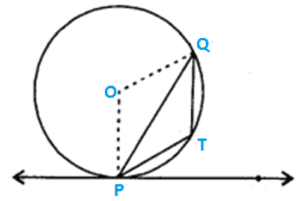Solution.

Since OP is perpendicular to PT.

∠OPT = 90°

∠OPQ = 90°- ∠QPT

∠OPQ = 90 – 60 = 30°.

In ΔOPQ, OP= OQ = r  ( Radius of the circle )

∠OPQ= ∠OQP = 30.

And,

∠POQ = 180 – ∠OPQ- ∠OQP

= 180° – 30° – 30° = 120°

Also, reflex ∠POQ = 360° – 120° = 240°

Now, ∠PRQ =  reflex ∠POQ

= 12x 240° = 120°

2. If the angle between two radii of a circle is 130°, then find the degree measure of the angle between the tangents at the ends of the radii.

Solution.

It is already known that angle between two radii and the angle between the tangents at the ends of the radii are supplementary.

Hence, Angle between the tangents at the ends of the radii is 180° – 130° , i.e., 50°.

3. In the figure, if ∠AOB = 125°, then find the degree measure of ∠

Solution.

It is already known that the opposite sides of a quadrilateral circumscribing a circle subtend supplementary angles at the centre of the circle.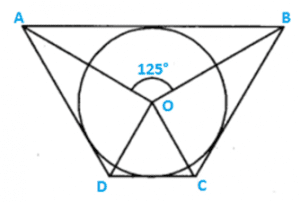∠AOB + ∠COD = 180°.

125° + ∠COD = 180°

∠COD = 180° – 125° = 55°.

4. In the figure, AB is a chord of the circle and AOC is its diameter such that ∠ACB = 50°. If AT is the tangent to the circle at the point A, then find the measure of ∠BAT.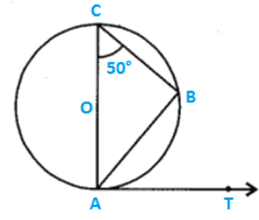Solution.

∠BAT= 50°.

5. At one end A of a diameter AB of a circle of radius 5cm, tangent XAY is drawn to the circle. Find the length of the chord CD parallel to XY and at a distance 8cm from A.

Solution.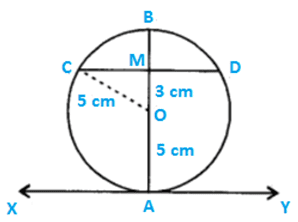Since XAY is a tangent through one end A of a diameter of a circle,

AB is perpendicular to XY

And also, CD is parallel to XAY ⇒ AB is perpendicular to CD

Since OM is perpendicular from centre O to the chord CD.

⤑ OM is perpendicular bisector of chord CD.

That is.,    CM = MD = 12CD.

Now, AM =8cm (given)

⇒  OM = AM – AO = 8-5 = 3cm.

In OMC, we obtain

CM = CM=OC2OM2$CM = sqrt{OC^{2}-OM^{2}}$

= 5232$sqrt{5^{2}-3^{2}}$

= 16$sqrt{16}$

= 4 cm.

Hence, CD = 2cm = 2 x 4 = 8 cm.

6. In the given figure, AT is a tangent to the circle with centre O such that OT = 4 cm and ∠OTA = 30°. Find the length of the segment AT.

Solution.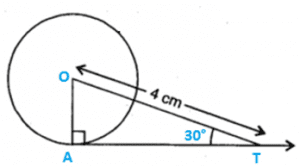In tight triangle ∆OTA, ∠OTA = 30°.

OAOT=sin30$frac{OA}{OT} = sin 30^{circ}$
OA=12OT=12×4=2cm$OA = frac{1}{2}OT = frac{1}{2} imes 4 = 2cm$

Again, AT=OT2OA2$AT = sqrt{OT^{2}-OA^{2}}$

AT = 4222$sqrt{4^{2}-2^{2}}$

=164$=sqrt{16-4}$
12$sqrt{12}$

= 23$2sqrt{3}$cm.

7. In the figure, if O is the centre of a circle, PQ is a chord and the tangent PR at P makes an angle of 50° with PQ, find the measure of ∠POQ.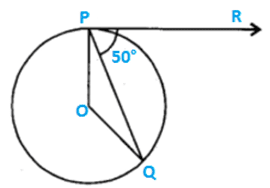Solution.

Since OP is perpendicular to PR

∠OPR = 90°

∠OPQ + ∠QPR = 90°

∠OPQ + 50° = 90°

→ ∠OPQ = ∠OQP = 40°

Again,

∠POQ + ∠OPQ  + ∠OQP = 180°

→ ∠POQ + 40° + 40° = 180°

→ ∠POQ = 180° – 40° – 40°

= 100°

8. In the figure, if PA and PB are tangents to the circle with centre O such that ∠APB = 50°, find the degree measure of ∠OAB.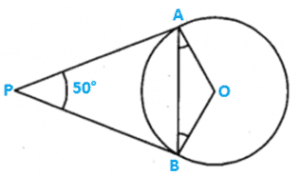Solution.

Since OA is perpendicular to PA and also, OB is perpendicular to PB

∠APB + ∠AOB = 180°

50°+ ∠AOB = 180°

∠AOB = 180° –  50° = 130°

In △AOB,

OA = OB = radii of same circle

∠OAB = ∠OBA = x ( say )

Again, ∠OAB + ∠OBA + ∠AOB = 180°

x +x + 130° = 180°

2x = 180° –  130° = 50°

X = 25°

Hence, ∠OAB =25°

9. In the figure, if PQR is the tangent to a circle at Q whose centre is O, AB is a chord parallel to PR and ∠BQR = 70°, find the measure of ∠AQB.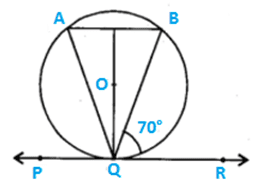Solution.

Since AB is parallel to PQR

∠B =∠BQR

→ ∠B =70°

Also, ∠A = ∠BQR    [∠s in the corresponding alternate segments are equal ]

→ ∠A = 70°

Now, in △ABQ, we have

∠A + ∠B + ∠AQB = 180°

70° + 70° + ∠AQB = 180°

∠AQB=180° – 70° – 70°

=40°

Hence,  ∠AQB = 40°

10. In the figure, DE and DF are tangents from an external point D to a circle with centre A. If DE=5cm and DE is perpendicular DF, find the radius of the circle.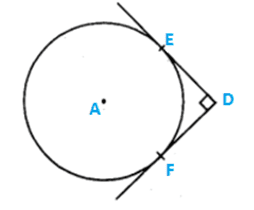Solution.

Join AE and AF → AE is perpendicular to DE and AF is perpendicular to DF.

AEDF is a square of side 5cm [DE = 5cm(given)]

Hence, radius of the circle is 5 cm.

11. If two tangents inclined at an angle of 60° are drawn to a circle of radius 3cm, find the length of each tangent.

Solution.

.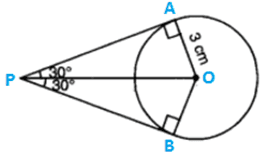Here, let PA and PB be two tangents to a circle with centre O.

OA is perpendicular to AP.

Since OP bisects ∠APB

∠OPA = 30°

Now, in △OAP, we have

APOA=cot30$frac{AP}{OA} = cot 30^{circ}$

→  AP3=3$frac{AP}{3} = sqrt{3}$

AP=33cm$AP = 3sqrt{3}cm$

12. From a point P which is at a distance of 13cm from the centre O of a circle of radius 5cm, a pair of tangents PQ and PR to the circle are drawn. Find the area of the quadrilateral PQOR.

Solution.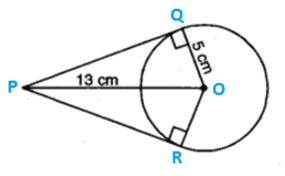In △OPQ, we have

OQ perpendicular  to PQ

OP2=PQ2+OQ2$OP^{2} = PQ^{2}+OQ^{2}$
132=PQ2+52$13^{2} = PQ^{2}+5^{2}$

PQ2=16925$PQ^{2} = 169 – 25$ = 144

PQ = 12cm

Now, (△OPQ) = x PQxOQ

= x12x5 = 30cm2$cm^{2}$

Thus, (quad, PQOR) = 2 x ar(△OPQ)

= 2×30 cm2$cm^{2}$

= 60cm2$cm^{2}$

NCERT solutions class 10 maths chapter 10 pdf are prepared by our subject experts under the guidelines of NCERT to assist students in their board exam preparations.

Circles is a part of Geometry, which is considered to be one of the major topic usually asked in the examination. Students, who aim to secure excellent result need to have a good understanding of this chapter 10 – circles. Circles basically consist of various terminologies, such as radius, diameter, chords, segment, angles etc. and its properties that needs to be understood for solving various questions on circles. These theorems and formulas are easy to remember and also can also be proven. The students preparing for the board examination must have a good practice of this circles chapter, as many questions can be framed from this chapter. Having a good practice of this chapter helps students to improve their 10th class maths result in board examination.

Students can find a good number of questions from this chapter in pdf, thus one should have a good practice of circles. Circles holds various important theorems in mathematics and it should be thoroughly practiced. Thus we at BYJU’S provide students with the NCERT Solution for class 10 Maths chapter 10, Circles for practice. With good practice, students can get to know all the method of solving the questions of different kind as well as the various important part from which students can get the question. Thus practicing questions and reviewing the various important questions back from the textbook can help students to have a better way of solving questions. Students preparing for the Board Examination can get all the Solution for NCERT chapter 10 circles.